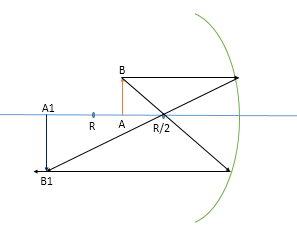# Consider a concave mirror with radius R. An upright object is placed in between the interval R/2...

## Question:

Consider a concave mirror with radius R. An upright object is placed in between the interval R/2 and R. What is the nature of the image?

(a) virtual, inverted, enlarged.

(b) real, inverted, reduced.

(c) virtual, upright, enlarged.

(d) real, inverted, enlarged.

(e) virtual, upright, reduced.

(f) virtual, inverted, reduced.

(g) virtual, upright, same size.

(h) real, upright, reduced.

(i) real, upright, same size.

(j) real, upright, enlarged.

## Reflection:

Reflection is the phenomenon in which light after reflecting from a shiner and opaque surface gets reflected and the law of reflection says the angle made by the incident ray to the normal will be equal to the angle of reflection between the normal and the reflected ray.

Given

The radius of curvature of the mirror is {eq}R {/eq}

Now the image formed is shown in the figure:Here the image formed is real and inverted on the same side where the object is placed.

The Image formed is bigger than the object.

Thus, the correct option is (d) and the image formed is real, inverted and enlarged.